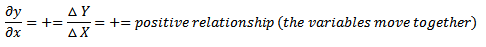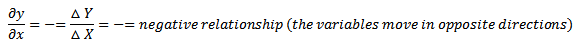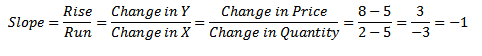All you need to know about graphs for introductory economics - FreeEconHelp.com, Learning Economics... Solved!

## 8/26/11

1)            Graphs of one variable, include bar graphs and pie charts.  They show information/facts, give a description of what is.  For example, bar graphs will have a number/variables on the y axis, and several distinct components on the x axis.  The value of each component on the x axis is measured in the single variable shown on the y axis.  A pie chart has a similar approach (except the variable is always a percentage and should add to 100%).

Another example is a time series graph, where the x axis shows different periods of time, but these are
still only measured with one variable that is shown on the y axis (possibly a dollar value, or temperature, etc.).

2)            Graphs of two variables, graphs where you can potentially see relationships between variables.  The most common example in economics is a graph with quantity on the x axis, and price on the y axis.

 Price (dollars per broccoli) Quantity (broccoli per month) 9 1 8 2 7 3 6 4 5 5 4 6 3 7 2 8 1 9

A graph showing the relationship between price and quantity, which is downward sloping gives us the demand curve.

3)            With this data and the resulting curve, you can figure out the relationship between the two variables.  In general you will have three different types of relationships: positive, negative, or no relationship.

A positive relationship means that when one variable increases, so does the other.  This means you will have an upward sloping curve, and a positive slope.A negative relationship means that when one variable increases, the other decreases.  This means you will have a downward sloping curve, and a negative slope.Finally, you could have no relationship between the two variables.  This would happen if you have a completely vertical or horizontal line (indicating no change in one variable).  Or random dots throughout the graph with no real trend up or down.

4)            How to find the slope of a line (moving from a price of 8 to 5):5)            What if the graph has more than two variables?
The demand curve graph drawn above was created considering everything else in the world constant.  What happens if something outside of the graph (NOT quantity or price) changes, such as income?  Generally, we would think that if income went up, we would demand more of a product, so we could see a shift in the demand curve.  Imagine that if income went up, we would demand one more broccoli at every price level.  The table would now look like:

 Price (dollars per broccoli) Quantity Income up Quantity Income Normal Quantity Income down 9 2 1 0 8 3 2 1 7 4 3 2 6 5 4 3 5 6 5 4 4 7 6 5 3 8 7 6 2 9 8 7 1 10 9 8

Where D2 shows the case where income goes up, and D3 shows the case where income goes down.
One of the benefits of using graphs is being able to tell a story about their relationship.  For example, if we were to draw a graph relating income to spending, we would probably see a positive relationship, or an upward sloping line.  This would be a good candidate for telling a story, and linking the two variables as a cause and effect.  It makes sense that as incomes rise that we will see more spending going on.

However, if we were to graph the number of Dalmatians that were being adopted in London, which unemployment rates in the US we may see a positive relationship between the two variables.  However, is it legitimate to tell a cause and effect story between these two variables?  No, they are not related, and we see a spurious correlation between the two (meaning that there is no meaningful link).
Also be on the lookout for the problem of reverse causality.  This problem occurs when X causes Y, but we conclude that Y causes X.  Let’s look out our income and spending example again.  We told the story that a rise in income would lead to rise in spending, but if we were to graph the relationship we could easily come to the conclusion that a rise in spending led to a rise in income.  However, if we think about it and observe what is going on in people’s minds, we see that it is the rise in income that causes a rise in spending and not the other way around.

6)            Calculating an area of a triangle:

Calculating an area of a rectangle:

Calculating slopes if your curves are not linear.
Draw a tangent line (a line that has the same curve as a point on the non-linear curve) and figure out the slope of that linear curve.  This obviously only gives you the slope at one point, but if you were to do this enough times you would get the slope of the entire curve.  This approach is useful when trying to figure out the change in slopes over the length of a curve.

You can see that the slopes of the two curves are different, with the second curve having the steeper slope (more negative in value).